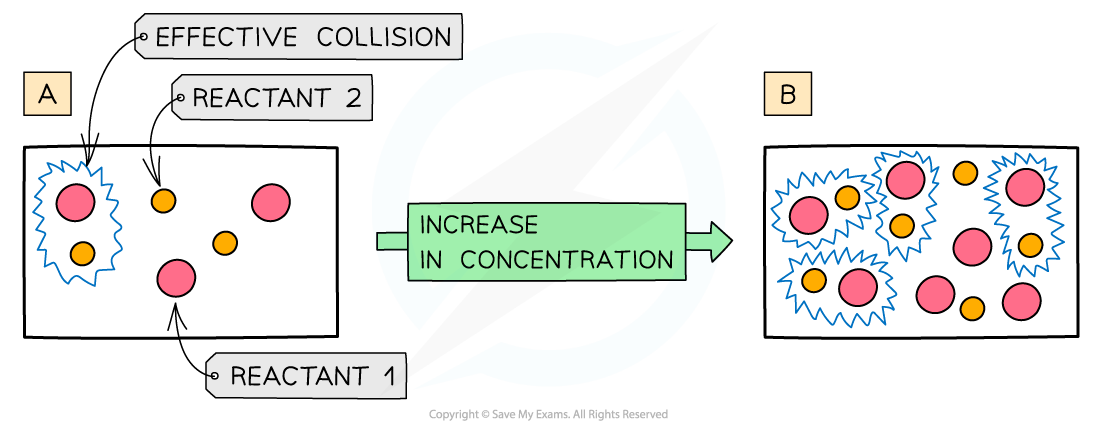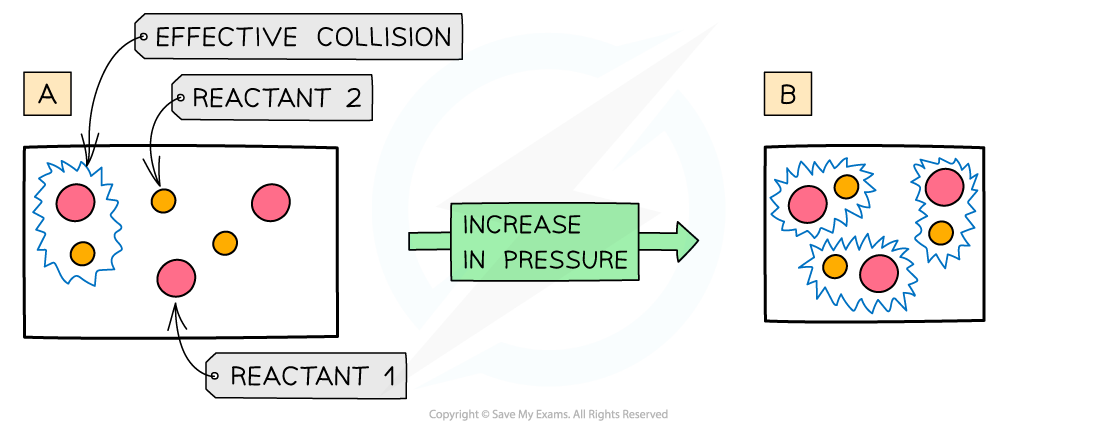# AQA A Level Chemistry复习笔记1.7.5 Effect of Concentration & Pressure

### Concentration, Pressure & Rate of Reaction

#### Concentration

• The more concentrated a solution is, the greater the number of particles in a given volume of solvent
• An increase in concentration causes in an increased collision frequency and therefore an increased rate of reactionThe diagram shows a higher concentration of particles in (b) which means that there are more particles present in the same volume than (a) so the chances and frequency of collisions between reacting particles is increased causing an increased rate of reaction

#### Pressure

• An increase in pressure in reactions that involve gases has the same effect as an increase in the concentrations of solutions
• When the pressure is increased, the molecules have less space in which they can move
• This means that the number of effective collisions increases due to an increased collision frequency
• An increase in pressure therefore increases the rate of reactionThe diagram shows a higher pressure in (b) which means that the same number of particles occupy a smaller volume, resulting in an increased collision frequency and therefore increased rate of reaction